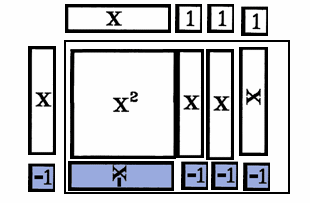The Visual / Auditory / Symbolic / Kinesthetic Approach to Algebra

Term Tiles are really only a support tool for each of these topics.

 Find A Common Denominator.

Finding a common denominator, if not done mentally or quickly, is a pain in the neck, and is used in only two instances in basic arithmetic and algebra. It is used to add or subtract fractions, and to simplify equations containing varied fractions.

Earlier an alternate but traditional method, "Add Fractions," was examined which obviates finding a common denominator. There isn't really an alternate method for solving equations containing many fractions.

Term Tiles & Tokens DON'T lend themselves to factoring into more than two expressions, so the best that can be suggested is a blend of paper and pencil work and manipulative work. Factor mentally or with Term Tiles. Record the prime factorization with pencil and paper.

Paper and pencil computation of common denominators may be found at example 7.

Find A Common Denominator
 1st: Factor each denominator writing the denominator as its prime factorization. 2nd: List the common factors, the factors each fraction shares, writing that factor only once. 3rd: Add to the list the factors each fraction does not share, listing only once the ones they have in common with some of the other fractions. 4th: The list of factors is the common denominator. Multiply the factors together if needed.The first fraction has denominator factors of x+1 and x-1. The second fraction's denominator is (x+1)(x+3). The x+1 is a duplicate. The x+3 and x-1 are unique. List these 3 factors. The common denominator is (x+1)(x-1)(x+3).

 Solve An Equation with Fractions.

To solve an algebraic proportion, see "Solve A Proportion," don't bother with this technique, even though it works.

To solve any other algebraic equation with fractions, multiply each fraction by the common denominator of the equation, then check for extraneous roots.

Solve.

 4 + 1 4 = xý-1 xý+4x+3 xý+2x-3

Finding the common denominator of the equation requires one to factor each denominator.

The first two denominators were factored above. Factor the last denominator mentally. It is factored with Tiles below at the right.4 + 1 4 = xý-1 xý+4x+3 xý+2x-3 (x+1)(x-1) (x+1)(x+3) (x+3)(x-1)

The common denominator is (x+1)(x-1)(x+3).

Multiply each fraction by the common denominator, reducing each fraction in tern and writing only the simplifications.4(x+3) + 1(x-1) = 4(x+1)

Solve and check the solution.

x = -7www.termtiles.com, Unit 36   © 2008, A. Azzolino